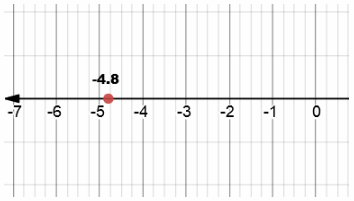# Texas Go Math Grade 6 Lesson 2.2 Answer Key Identifying Opposites and Absolute Value of Rational Numbers

Refer to our Texas Go Math Grade 6 Answer Key Pdf to score good marks in the exams. Test yourself by practicing the problems from Texas Go Math Grade 6 Lesson 2.2 Answer Key Identifying Opposites and Absolute Value of Rational Numbers.

## Texas Go Math Grade 6 Lesson 2.2 Answer Key Identifying Opposites and Absolute Value of Rational Numbers

Reflect

Question 1.
Communicate Mathematical Ideas How would you graph – 2.25? Would it be left or right of point D?
I would graph number – 2.25 by splitting distance between numbers – 2 and – 3 into 4 parts!
Than i would pick the first one on right and that would be number – 2.25
Number – 2.25 is on right of D.

Question 2.
What are the opposites of 7, – 3.5, 2.25, and 9$$\frac{1}{3}$$?
Opposites of numbers, as we learned in previous section, are those same numbers with added – sign!
So, opposites of 7, – 3.5, 2.25, 9$$\frac{1}{3}$$ are numbers – 7, 3.5, 2.25, – 9$$\frac{1}{3}$$ respectively!

Reflect

Question 3.
Communicate Mathematical Ideas What is the absolute value of the average January low temperature in 2011? How do you know?Distance from 3.8 to 0 is 3.8 units!
Therefore, its absolute value is 3.8

Graph each number on the number line. Then use your number line to find each absolute value.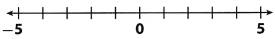Question 4.
– 4.5; |- 4.5| = __________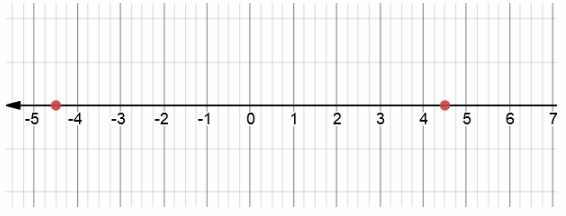As i mentioned before, absolute value of negative number is positive number!
As its absolute value, it must have same distance from 0 as number, so solution is 4.5

Question 5.
1$$\frac{1}{2}$$; |1$$\frac{1}{2}$$| = __________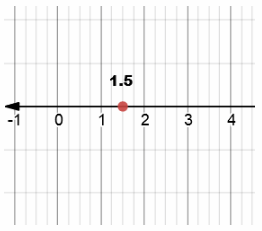Note that i used 1.5 instead of 1$$\frac{1}{2}$$ as i can’t write that expression on graph.
As i mentioned before, absolute value of positive number is same number!
As its absolute value, it must have same distance from 0 as number,
so solution is 1$$\frac{1}{2}$$

Question 6.
4; |4| = __________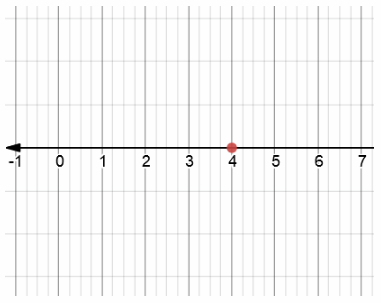As i mentioned before, absolute value of positive number is same number!
As its absolute value, it must have same distance from 0 as number,
so solution is 4

Question 7.
– 3$$\frac{1}{4}$$; |- 3$$\frac{1}{4}$$| = __________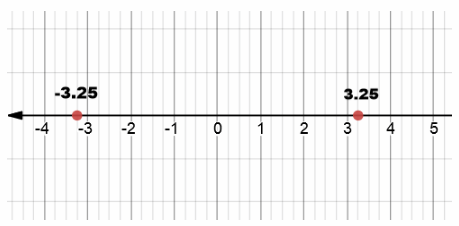Note that i used 3.25 instead of 3$$\frac{1}{4}$$
As i mentioned before, absolute value of negative number is positive number!
As its absolute value, it must have same distance from 0 as number,
so solution is 33$$\frac{1}{4}$$

Graph each number and Its opposite on a number line.

Question 1.
– 2.8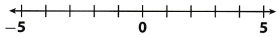Opposite of – 2.8 is 2.8Question 2.
4.3Opposite of 4.3 is – 4.3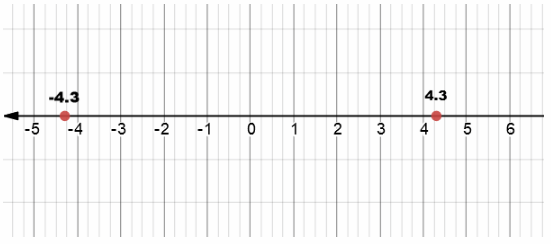Question 3.
– 3$$\frac{4}{5}$$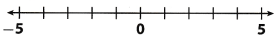Opposite of – 3$$\frac{4}{5}$$ is 3$$\frac{4}{5}$$Note that i used 3.8 instead of 3$$\frac{4}{5}$$ in my graph!

Question 4.
1$$\frac{1}{3}$$Opposite of 1$$\frac{1}{3}$$ is – 1$$\frac{1}{3}$$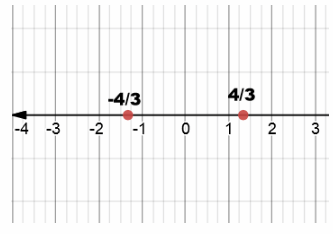Note that i used 4/3 instead of 1$$\frac{1}{3}$$ on my graph.

Find the opposite of each number.

Question 5.
3.78 ______________
As i mentioned before, opposite of any number is number with added – sign on left side!
Opposite of 3.78 is – 3.78

Question 6.
– 7$$\frac{5}{12}$$ ______________
As i mentioned before, opposite of any number is number with added – sign on left side!
Opposite of –$$\frac{89}{12}$$ is $$\frac{89}{12}$$
Note that we used – $$\frac{89}{12}$$ instead of given expression, but they are equivalent.

Question 7.
0 ______________
As i mentioned before, opposite of any number is number with added – sign on left side!
Opposite of 0 is 0.

Question 8.
4.2 ______________
As i mentioned before, opposite of any number is number with added – sign on left side!
Opposite of 4.2 is – 4.2.

Question 9.
12.1 ______________
As i mentioned before, opposite of any number is number with added – sign on left side!
Opposite of 12.1 is – 12.1.

Question 10.
2.6 ______________
As i mentioned before, opposite of any number is number with added – sign on left side!
Opposite of 2.6 is – 2.6.

Question 11.
Vocabulary Explain why 2.15 and – 2.15 are opposites.
Numbers 2.15 and – 2.15 are opposites because they have same distances from 0 but are placed on different sides of 0.

Find the absolute value of each number.

Question 12.
5.23 ______________
We define absolute value as distance from 0. So, it is surely positive number.
Now, when we know this, we can conclude that absolute value of positive number is that exact same number, and absolute value of negative number is its opposite!
|5.23| = 5.23

Question 13.
– 4$$\frac{2}{11}$$ ______________
We define absolute value as distance from 0. So, it is surely positive number.
Now, when we know this, we can conclude that absolute value of positive number is that exact same number, and absolute value of negative number is its opposite!
|-$$\frac{46}{11}$$ | = $$\frac{46}{11}$$
Note that we used – $$\frac{46}{11}$$ instead of given expression, but they are equivalent!

Question 14.
0 ______________
We define absolute value as distance from 0. So, it is surely positive number.
Now, when we know this, we can conclude that absolute value of positive number is that exact same number, and absolute value of negative number is its opposite!
|0| = 0

Question 15.
– 6$$\frac{3}{5}$$ ______________
We define absolute value as distance from 0. So, it is surely positive number.
Now, when we know this, we can conclude that absolute value of positive number is that exact same number, and absolute value of negative number is its opposite!
|- 6$$\frac{3}{5}$$ | = 6$$\frac{3}{5}$$

Question 16.
– 2.12 ______________
We define absolute value as distance from 0. So, it is surely positive number.
Now, when we know this, we can conclude that absolute value of positive number is that exact same number, and absolute value of negative number is its opposite!
|- 2.12| = 2.12

Question 17.
8.2 ______________
We define absolute value as distance from 0. So, it is surely positive number.
Now, when we know this, we can conclude that absolute value of positive number is that exact same number, and absolute value of negative number is its opposite!
|8.2| = 8.2

Essential Question Check-In

Question 18.
How do you identify the opposite and the absolute value of a rational number?
Opposite of Rational number is the same distance on a number line from 0 but on the other side of 0 on a number line.

We define absolute value as distance from 0 on a number line
This definition is correct with Rational numbers
If a given number is negative, than its absolute value is its opposite!
If a given number is positive, than its absolute value is exact same number!

Question 19.
Financial Literacy A store’s balance sheet represents the amounts customers owe as negative numbers and credits to customers as positive numbers.a. Write the opposite of each customer’s balance.
Number 85.23 is opposite of – 85.23.
Number – 20.44 is opposite of 20.44.
Number 116.33 is opposite of – 116.33.
Number – 13.50 is opposite of 13.50.
Number – 9.85 is opposite of 9.85.

b. Mr. Yuan wants to use his credit to pay off the full amount that another customer owes. Which customer’s balance does Mr. Yuan have enough money to pay off?
Mr.Yuan can only pay for Wenner because he owes $9.85 and Mr. Yuan has credit of$13.50

c. Which customer’s balance would be farthest from 0 on a number line? Explain.
If we draw the number line, farthest would be Stein.
Absolute value of his balance is highest (- \$116.33).

Question 20.
Multistep Trina and Jessie went on a vacation to Hawaii. Trina went scuba diving and reached an elevation of – 85.6 meters, which is below sea level. Jessie went hang-gliding and reached an altitude of 87.9 meters, which is above sea level.

a. Who is closer to the surface of the ocean? Explain.
Here, we calculate absolute values of both given numbers:
|- 85.6| = 85.6
|87.9| = 87.9
Now, as we know that 85.6 is less than 87.9, that implies:
Trina is closer to ocean surface.

b. Trina wants to hang-glide at the same number of meters above sea level as she scuba-dived below sea level. Will she fly higher than Jessie did? Explain.
She won’t fly higher than Jessie did. Why?
We know that she would like to hang-glide 85.6 meters, which is lower than 87.9 meters.

Question 21.
Critical Thinking Carlos finds the absolute value of – 5.3, and then finds the opposite of his answer. Jason finds the opposite of – 5.3, and then finds the absolute value of his answer. Whose final value is greater? Explain.
Carlos firstly found absolute value of – 5.3 which is 5.3
Then he found opposite of 5.3 which is – 5.3
So his final value is: – 5.3

Jason firstly found opposite of – 5.3 which is 5.3.
Then he found absolute value of 5.3 which is 5.3
So his final value is: 5.3

Since 5.3 is greater than – 5.3, we can conclude that Jason’s final value is greater
Jason’s number is greater!

Question 22.
Explain the Error Two students are playing a math game. The object of the game is to make the least possible number by arranging the given digits inside absolute value bars on a card. In the first round, each player will use the digits 3, 5, and 7 to fill in the card.a. One student arranges the numbers on the card as shown. What was this student’s mistake?
This student didn’t find Least possible number.
He miss used operation of absolute value.
The key point was to find a number which is closer to 0, but he didn’t get the point!

b. What is the least possible number the card can show?
Least possible number that card can show is – 3.57

H.O.T. Focus On Higher Order Thinking

Question 23.
Analyze Relationships If you plot the point – 8.85 on a number line, would you place it to the left or right of – 8.8? Explain.
Number – 8.85 is smaller than – 8.80.
Therefore, number – 8.85 would be placed on Left of – 8.80

Question 24.
Make a Conjecture If the absolute value of a negative number is 2.78, what is the distance on the number line between the number and its absolute value? Explain your answer.
Firstly, we calculate absolute value of 2.78:
|2.78| = 2.78
As given number is – 2.78, to calculate distance to its absolute value:
We must multiply its positive value by 2 because we have same distances from both sides of 0 on a number Line.

Result: 5.56

Question 25.
Multiple Representations The deepest point in the Indian Ocean is the Java Trench, which is 25,344 feet below sea level. Elevations below sea level are represented by negative numbers.

a. Write the elevation of the Java Trench.
If we know that deepest point is 25. 344 feet beLow sea leveL.
Than we can concLude that our vaLue wiLL be negative.
Therefore, eLevation of Java Trench is: – 25. 344 feet

b. A mile is 5,280 feet. Between which two integers is the elevation in miles?
As given. 1 mile equals 5,280 feet. So to get elevation in miles, We have to convert our value into miles!

We get: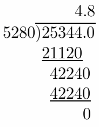Now we know that elevation of Java Trench is – 4.8 miles

So, now it is obvious to conclude that elevation of – 4.8 miles lies on a number line between integers – 4 and – 5.

c. Graph the elevation of the Java Trench in miles.